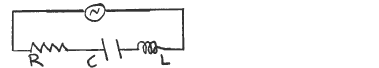# Problem: In an R-L-C ac series circuit the voltage across the capacitor is 64 V and the voltage amplitude across the inductor is 96 V. Does the source voltage lag or lead the current in the circuit?(a) lag(b) lead

81% (46 ratings)
###### Problem Details

In an R-L-C ac series circuit the voltage across the capacitor is 64 V and the voltage amplitude across the inductor is 96 V. Does the source voltage lag or lead the current in the circuit?

(a) lag

(b) leadFrequently Asked Questions

What scientific concept do you need to know in order to solve this problem?

Our tutors have indicated that to solve this problem you will need to apply the Impedance in AC Circuits concept. You can view video lessons to learn Impedance in AC Circuits. Or if you need more Impedance in AC Circuits practice, you can also practice Impedance in AC Circuits practice problems.

How long does this problem take to solve?

Our expert Physics tutor, Juan took 1 minute and 48 seconds to solve this problem. You can follow their steps in the video explanation above.